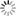# Episode List

OR

### Season 2

14 Sep. 1952
Holiday SongBe the first one to add a plot.

28 Sep. 1952
The RoomBe the first one to add a plot.

12 Oct. 1952
O RomanyBe the first one to add a plot.

26 Oct. 1952
Better Than WalkingBe the first one to add a plot.

9 Nov. 1952
The Darkness BelowBe the first one to add a plot.

23 Nov. 1952
The Old BeginningBe the first one to add a plot.

7 Dec. 1952
The SearchBe the first one to add a plot.

21 Dec. 1952
Mr. Quimby's Christmas HatsBe the first one to add a plot.

1 Feb. 1953
A Medal in the FamilyBe the first one to add a plot.

22 Feb. 1953
Wings on My FeetBe the first one to add a plot.

8 Mar. 1953
The RumorBe the first one to add a plot.

15 Mar. 1953
The GestureBe the first one to add a plot.

29 Mar. 1953
Wish on the MoonBe the first one to add a plot.

12 Apr. 1953
The Long Way HomeBe the first one to add a plot.

26 Apr. 1953
Printer's MeasureAn old man is proud of his skill as a printer and resents the way new technology is likely to make him obsolete.

3 May 1953
The AccidentBe the first one to add a plot.

17 May 1953
The Oil WellBe the first one to add a plot.

31 May 1953
Before I WakeBe the first one to add a plot.

14 Jun. 1953
Her Prince CharmingA lonely old woman who spends all her time going to radio and TV quiz shows wins a weekend as the guest of an old time movie star.

28 Jun. 1953
Catch a Falling StarBe the first one to add a plot.

12 Jul. 1953
Nothing to Sneeze AtBe the first one to add a plot.

26 Jul. 1953
The Young and the FairBe the first one to add a plot.

9 Aug. 1953
Ernie Barger Is 50Be the first one to add a plot.

23 Aug. 1953
Hollywood Tandem